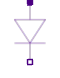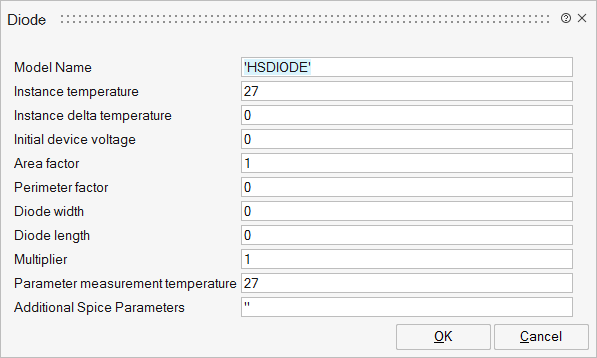# Diode

Simple DiodeAnalog/Basic

## Description

The simple diode is a two-terminal electronic (Anode and Cathode) component that conducts current which, passing through a junction, can only go in one direction, called the forward direction.

Current trying to flow the reverse direction is blocked.

It consists of the diode itself and an parallel ohmic resistance R. The diode formula is:If the exponent v/Vt reaches the limit maxex, the diode characteristic is linearly continued to avoid overflow.

Please note: In case of useHeatPort=true the temperature dependence of the electrical behavior is not taken into account yet. The parameters are not temperature dependent.

## ParametersNameLabelDescriptionData TypeValid Values

sp_mname

Model Name

For more details, see Model names.

String

sp_temp

Instance temperature

The temperature at which the diode is operating (degrees Celcius)

Scalar

sp_dtemp

Instance delta temperature

Temperature variation relative to the simulation temperature (degrees Celcius)

Scalar

sp_ic

Initial device voltage

Initial diode voltage (volts)

Scalar

sp_area

Area factor

Junction area used to scale any pn junction (square meter).

The model parameters IS, CJ0, RS and IBV are all proportional to device area.

Scalar

sp_pj

Perimeter factor

Perimeter factor

Scalar

sp_w

Diode width

Junction width (meter)

Scalar

sp_l

Diode length

Junction length (meter)

Scalar

sp_m

Multiplier

Scale factor

Scalar

sp_tnom

Parameter measurement temperature

Instance operating temperature (degrees Celsius)

Scalar

sp_Spice

For more details, see Additional Spice Parameters.

String

## Ports

NameTypeDescriptionIO TypeNumber

D+

implicit

Anode - Positive pin

1

D-

implicit

Cathode - Negative pin

2